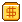# 生活不易、且行且珍惜。网站首页技术分享

## Java中的BigDecimal详解

今天碰到一个问题，金额计算用double类型会丢失经度，就改用了BigDecimal类型，这个类型之前用的比较少，没怎么接触。就到网上看了一下相关教程，写个总结记一下。

BigDecimal类

对于不需要任何准确计算精度的数字可以直接使用float或double，但是如果需要精确计算的结果，则必须使用BigDecimal类，而且使用BigDecimal类也可以进行大数的操作。

## BigDecimal构造方法

1.public BigDecimal(double val)    将double表示形式转换为BigDecimal

2.public BigDecimal(int val)　　将int表示形式转换成BigDecimal

3.public BigDecimal(String val)　　将String表示形式转换成BigDecimal

测试

```System.out.println(new BigDecimal(0.1).toString());
System.out.println(new BigDecimal("0.1").toString());
System.out.println(new BigDecimal(Double.toString(
0.1000000000000000055511151231257827021181583404541015625)).toString());
System.out.println(new BigDecimal(Double.toString(0.1)).toString());```

输出结果

```// 0.1000000000000000055511151231257827021181583404541015625
// 0.1
// 0.1
// 0.1```

分析：

第一行：事实上，由于二进制无法精确地表示十进制小数0.1，但是编译器读到字符串"0.1"之后，必须把它转成8个字节的double值，因此，编译器只能用一个最接近的值来代替0.1了，即0.1000000000000000055511151231257827021181583404541015625。因此，在运行时，传给BigDecimal构造函数的真正的数值是0.1000000000000000055511151231257827021181583404541015625。

第二行：BigDecimal能够正确地把字符串转化成真正精确的浮点数。

第三行：问题在于Double.toString会使用一定的精度来四舍五入double，然后再输出。会。Double.toString(0.1000000000000000055511151231257827021181583404541015625)输出的事实上是"0.1"，因此生成的BigDecimal表示的数也是0.1。

第四行：基于前面的分析，事实上这一行代码等价于第三行

结论：

1.如果你希望BigDecimal能够精确地表示你希望的数值，那么一定要使用字符串来表示小数，并传递给BigDecimal的构造函数。

2.如果你使用Double.toString来把double转化字符串，然后调用BigDecimal(String)，这个也是不靠谱的，它不一定按你的想法工作。

3.如果你不是很在乎是否完全精确地表示，并且使用了BigDecimal(double)，那么要注意double本身的特例，double的规范本身定义了几个特殊的double值(Infinite，-Infinite，NaN)，不要把这些值传给BigDecimal，否则会抛出异常。

JDK的描述：1、参数类型为double的构造方法的结果有一定的不可预知性。有人可能认为在Java中写入newBigDecimal(0.1)所创建的BigDecimal正好等于 0.1（非标度值 1，其标度为 1），但是它实际上等于0.1000000000000000055511151231257827021181583404541015625。这是因为0.1无法准确地表示为 double（或者说对于该情况，不能表示为任何有限长度的二进制小数）。这样，传入到构造方法的值不会正好等于 0.1（虽然表面上等于该值）。

2、另一方面，String 构造方法是完全可预知的：写入 newBigDecimal("0.1") 将创建一个 BigDecimal，它正好等于预期的 0.1。因此，比较而言，通常建议优先使用String构造方法

double必须用作BigDecimal的源时，请使用`Double.toString(double)``转成String，然后`使用String构造方法，或使用BigDecimal的静态方法valueOf

```public static void main(String[] args)
{
BigDecimal bDouble1 = BigDecimal.valueOf(2.3);
BigDecimal bDouble2 = new BigDecimal(Double.toString(2.3));

System.out.println("bDouble1=" + bDouble1); //2.3
System.out.println("bDouble2=" + bDouble2); //2.3

}```

把double强制转化成int

`int x=(int)1023.99999999999999; // x=1024 为什么？`

原因还是在于二进制无法精确地表示某些十进制小数，因此1023.99999999999999在编译之后的double值变成了1024。

所以，把double强制转化成int确实是扔掉小数部分，但是你写在代码中的值，并不一定是编译器生成的真正的double值

验证代码：

```double d = 1023.99999999999999;
int x = (int) d;
System.out.println(new BigDecimal(d).toString()); // 1024
System.out.println(Long.toHexString(
Double.doubleToRawLongBits(d))); // 4090000000000000
System.out.println(x); // 1024```

# BigDecimal加减乘除运算

```public BigDecimal add(BigDecimal value);            //加法
public BigDecimal subtract(BigDecimal value);       //减法
public BigDecimal multiply(BigDecimal value);       //乘法
public BigDecimal divide(BigDecimal value);         //除法```

代码实例

```public static void main(String[] args)
{
BigDecimal a = new BigDecimal("4.5");
BigDecimal b = new BigDecimal("1.5");

System.out.println("a + b =" + a.add(b));  //6.0
System.out.println("a - b =" + a.subtract(b));  //3.0
System.out.println("a * b =" + a.multiply(b)); //6.75
System.out.println("a / b =" + a.divide(b));  //3
}```

这里有一点需要注意的是除法运算divide.

BigDecimal除法可能出现不能整除的情况，比如 4.5/1.3，这时会报错java.lang.ArithmeticException: Non-terminating decimal expansion; no exact representable decimal result.

其实divide方法有可以传三个参数

`public BigDecimal divide(BigDecimal divisor, int scale, int roundingMode) 第一参数表示除数， 第二个参数表示小数点后保留位数，第三个参数表示舍入模式，只有在作除法运算或四舍五入时才用到舍入模式，有下面这几种ROUND_CEILING    //向正无穷方向舍入ROUND_DOWN    //向零方向舍入ROUND_FLOOR    //向负无穷方向舍入ROUND_HALF_DOWN    //向（距离）最近的一边舍入，除非两边（的距离）是相等,  如果是这样，向下舍入, 例如1.55 保留一位小数结果为1.5                   ROUND_HALF_EVEN    //向（距离）最近的一边舍入，除非两边（的距离）是相等, 如果是这样，如果保留位数是奇数，使用                   ROUND_HALF_UP，如果是偶数，使用ROUND_HALF_DOWNROUND_HALF_UP    //向（距离）最近的一边舍入，除非两边（的距离）是相等,如果是这样，向上舍入, 1.55保留一位小数结果为1.6                      ROUND_UNNECESSARY    //计算结果是精确的，不需要舍入模式ROUND_UP    //向远离0的方向舍入`

按照各自的需要，可传入合适的第三个参数。四舍五入采用 ROUND_HALF_UP

需要对BigDecimal进行截断和四舍五入可用setScale方法，例：

```public static void main(String[] args)
{
BigDecimal a = new BigDecimal("4.5635");

a = a.setScale(3, RoundingMode.HALF_UP);    //保留3位小数，且四舍五入
System.out.println(a);
}```

`*减乘除其实最终都返回的是一个新的BigDecimal对象，因为BigInteger与BigDecimal都是不可变的（immutable）的，在进行每一步运算时，都会产生一个新的对象public static void main(String[] args)    {        BigDecimal a = new BigDecimal("4.5");        BigDecimal b = new BigDecimal("1.5");        a.add(b);        System.out.println(a);  //输出4.5. 加减乘除方法会返回一个新的BigDecimal对象                                  ，原来的a不变    }`

# 总结

(1)商业计算使用BigDecimal。（比如金额）

(2)尽量使用参数类型为String的构造函数。

(3) BigDecimal都是不可变的（immutable）的，在进行每一步运算时，都会产生一个新的对象，所以在做加减乘除运算时千万要保存操作后的值。

(4)我们往往容易忽略JDK底层的一些实现细节，导致出现错误，需要多加注意。

#去评论一下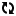+1

「万物皆有时，比如你我相遇」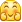发表
•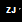•••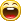•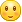•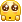••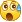•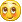•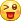••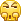•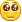••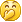•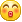••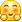•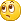•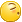•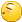••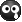•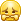•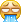•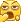••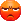•••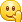•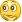•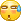•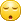•••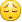••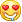•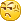•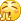•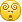•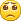•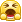•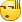•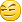•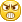•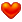•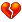•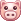•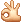•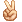•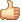•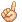•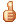••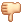•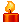••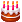••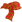•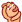•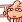••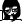•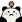•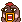•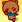•